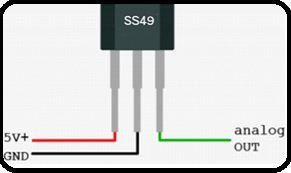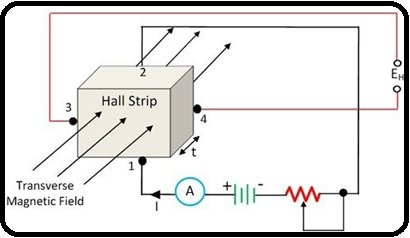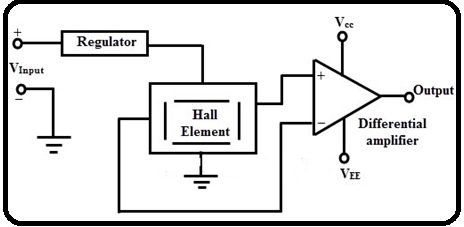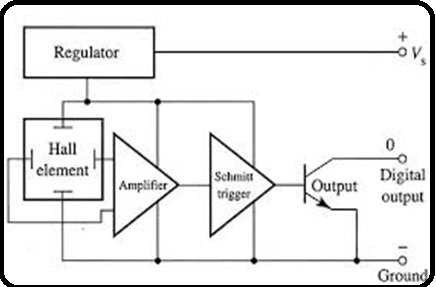# Hall effect sensor working, types and applications

### Introduction to Hall Effect Sensors

What is Hall Effect Sensor? In order to check the practical behavior of any electrical or electronic equipment, it is very necessary to install the device which communicate between this electrical or electronic equipment and your real time system. For this purpose, so many sensors and transducers are available in market which have been using for different purposes. But in this article, we would be only talk about the hall effect sensor. Hall effect sensor is the type of sensor which is typically used for detecting the practical behavior of anything such as motor, in response to changing magnetic field. Hall effect sensor is basically the transducers whose voltages varies by changing magnetic field. This sensor consists of a metal strip, when it is placed inside of any magnetic field then the presence of this magnetic field electron is deflected towards the edges of this strip then EMS is induced across its edges. In simple words it just works like as an analogue transducer. They are mainly used for speed detection, approximate switching, current and positioning sensing applications. A simple hall effect sensor is shown is figure 1 which consists of three terminals.Figure 1 Hall Effect Sensor

### Working Principle of Hall Effect Sensors

The working principle of hall effect sensor is very simple. It actually consists of a current carrying metal strip when this current carrying metal strip is placed inside any transverse magnetic field then EMF is developed across the edges of this current carrying metal strip. The magnitude of this developed EMF is depending upon the density of the flux and mobility of electron. This property of this current carrying conductor is called hall effect. This hall effect element is typically used for sensing current and magnetic field measurements. A hall effect element circuit is shown is figure 2.Figure 2 Hall Effect Sensor Circuit

According to the circuit when current is applied to terminal 1 and 3 then output is acquired at terminal 3 and 4. When no any magnetic field is applied to the strip then terminal 3 and 4 remains on same potential then there would be no any effect on hall effect strip. Similarly, when the magnetic field is applied on hall effect strip then EMF or voltages are induced across the terminal 3 and 4. These voltages are directly proportional to the material strength which have used for hall effect strip. These output voltages are obtained by the equation,

E = KHIB/t         (1)

Where KH is hall effect co-efficient which is obtained from equation 2

KH = v-m/A-wbm-2   (2)

Where KH is hall effect co-efficient , I is the current, B is the flux density in Wbm-2 and  t is thickness of strip.

Through the output voltages, current and magnetic field strength could be easily measured but to measure the hall effect EMF in a simple conductor is very difficult because it is very small. Instead of simple conductor if we used the germanium conductor then its hall effect EMF could be easily measured with the help of moving coil instrument.

### Types of Hall Effect Sensors

For making the useable of hall effect sensor output, it required a signal conditioning circuit. This circuit does the temperature compensation, amplification, linearity and voltage regulation etc. Currently two types of hall effect sensors have been used first one is analogue output hall effect sensor and second one is bi level or digital output hall effect sensor.

Analogue Hall Effect Sensor: If we compared this sensor with basic hall effect sensor then this type of sensor gives the stable behavior in noisy environment and have large voltage range to operate. An analogue hall effect sensor circuit is shown in figure 3, which gives the analogue output voltage which are proportional to exposed magnetic field.Figure 3 Analogue Hall Effect Sensor Circuit

In this circuit, differential amplifier provides the fixed off set or biased voltages, so that when there is no any magnetic field then these voltages are appeared across the output terminals of this circuit. These voltages are called null voltages.  At hall effect terminals, the magnetic field could be positive or negative. When the magnetic field is positive then output voltages is increased the null value voltages, similarly when magnetic field is negative then output voltage is decreased the null value voltages. These sensors are not so much accurate with magnetic field therefore these are required a proper calibration in that places where high precision measurements are required. The flexibility of this sensor is further  increased by adding the open emitter or open collector push pull transistor at the output side of differential amplifier.

Digital Output Hall Effect Sensor: This type of sensor has digital output means ON or OFF therefore this is also called bi level hall effect sensor. This sensor is almost same as analogue sensor but only the difference is that, there is an addiction of Schmitt trigger. In this Schmitt trigger, hysteresis thresh hold level is built in and this Schmitt trigger addiction assembly converts the analogue input into digital output after comparing differential amplifier output with reference fixed voltages. When the differential amplifier output is greater than fixed reference voltages then Schmitt trigger will be turned on similarly when differential amplifier output is less then fixed reference voltages then Schmitt trigger will be turned off. A simple digital output hall effect sensor is shown in figure 4Figure 4 Digital Output Hall Effect Sensor

### Different Applications of Hall Effect Sensor

Hall effect sensor has been used in different applications and its construction depends upon its configuration where it has been used. But these are mainly used in biomedical, automatic tellers, telecommunications, automobiles and process control industry.

Position Sensor: This type of hall effect sensor is used of sensing sliding motion and in this sensor, there is tightly gap between hall effect element and magnet. When the magnet is moved in forward and backward direction then magnetic field is produced. The polarity of this magnetic field is positive when the element is moved toward north pole similarly the magnetic field is positive when the element is moved toward south pole. These sensors are called approximate sensor and used for position sensing.

Brushless DC Motor Sensor: In brushless dc motors, power distribution is controlled through electronic commutation instead of mechanically commutation. For this purpose, three digital output hall effect sensors are installed at the stator end of brushless dc motor and for operating these sensors permanent magnet materials are installed at rotor shaft.

Current Sensor: It is the type of hall effect sensor which is used for measuring both ac and dc currents. These are available in range from 250mA to thousand amps. These are linear sensors and when this sensor is placed near magnetic field then voltage is developed across this sensor. The values of these voltages are proportional to magnetic field strength.

### 2 thoughts on “Hall effect sensor working, types and applications”

1. Very informative, Thank U